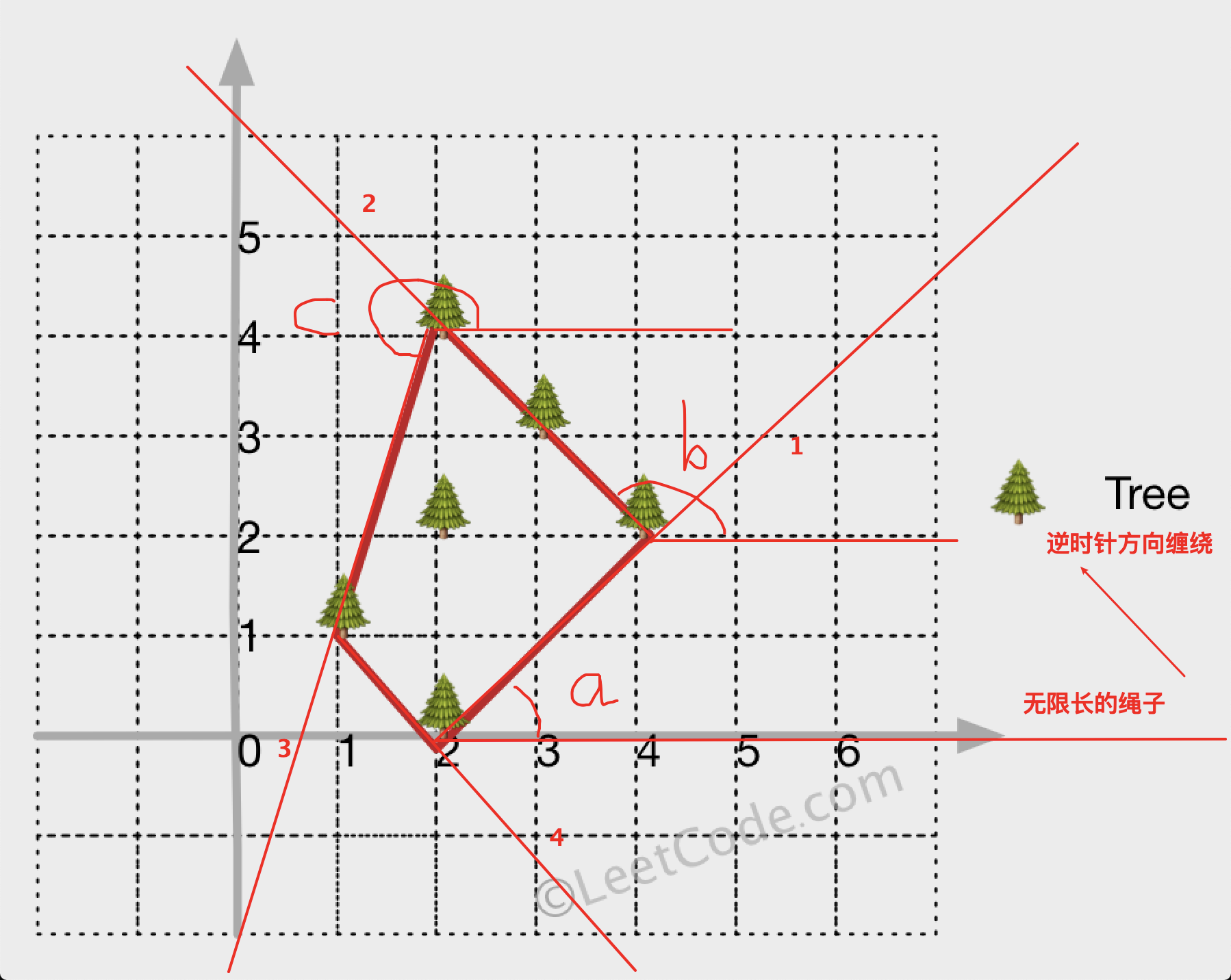# 587. 安装栅栏

``````class Solution {

public int[][] outerTrees(int[][] points) {
//list保存使用到的点的下标
List<Integer> list = new ArrayList<>();
int first = this.findFirst(points);//起点
int cur = first;//当前点
int next;//下一个点
//每次寻找的最小角度
double minAngle = 0;
do {
//找到的点加到结果中
if (!list.contains(cur)) {
}
//开始寻找下一个点
//下一个点的默认信息
next = first;
double[] nextPolar = new double[]{Double.MAX_VALUE, 2 * Math.PI};
//遍历每一个点
for (int i = 0; i < points.length; i++) {
if (cur==i) {
//当前点跳过
continue;
}
//计算每一个点基于当前点的极坐标
double[] polar = this.polarCoordinate(points[cur], points[i]);
if (polar >= minAngle) {
//满足最小角度的条件，取符合条件中角度最小，如果角度相等，取长度最短的值
if (polar < nextPolar || (polar == nextPolar && polar < nextPolar)) {
nextPolar = polar;
next = i;
}
}
}
//成功找到下一个点，更新最小角度
minAngle = nextPolar;
//更新当前点信息，继续循环找下一个点
cur = next;
} while (next != first);
//返回结果
int[][] res = new int[list.size()];
for (int i = 0; i < list.size(); i++) {
res[i] = points[list.get(i)];
}
return res;
}

/**
* 查找第一个节点，取所有值中y轴的值最小的点作为第一个节点
*
* @param points
* @return
*/
private int findFirst(int[][] points) {
int min = 0;
for (int i = 1; i < points.length; i++) {
if (points[i] < points[min]) {
min = i;
}
}
return min;
}

/**
* 获取p点基于o点的极坐标
* 返回一个数组，0为长度r，1为角度angle（用π标识的弧形角）
*
* @param o
* @param p
* @return
*/
private double[] polarCoordinate(int[] o, int[] p) {
double x = p - o;
double y = p - o;
//使用公式计算极坐标
double r = Math.sqrt(Math.pow(x, 2) + Math.pow(y, 2));
double angle = 0;
if (x > 0 && y >= 0) {
//第一象限
angle = Math.atan(y / x);
} else if (x < 0) {
//第二、三象限
angle = Math.atan(y / x) + Math.PI;
} else if (x > 0 && y < 0) {
//第四象限
angle = Math.atan(y / x) + Math.PI * 2;
} else if (x == 0 && y > 0) {
//y轴正轴
angle = Math.PI / 2;
} else if (x == 0 && y < 0) {
//y轴负轴
angle = Math.PI / 2 * 3;
} else if (x == 0 && y == 0) {
//原点
angle = 0;
}
return new double[]{r, angle};
}

}
``````